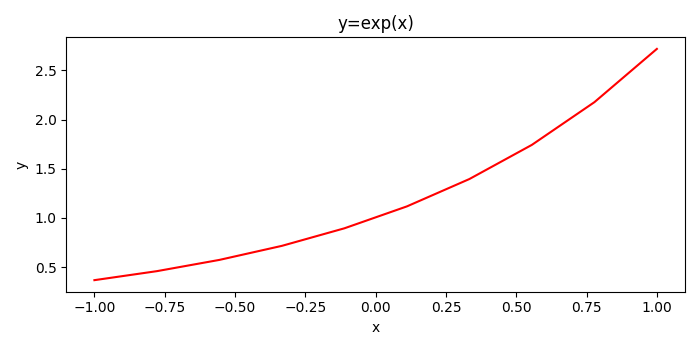# What is the preferred way to set Matplotlib figure/axes properties?

To set the properties of a plot, we can get the current axis of the plot. After that, we can perform several set_* methods to set the properties of the plot.

## Steps

• Create a figure and a set of subplots using subplots() method with figsize=(5, 5).

• Create x and y data points using numpy.

• Plot x and y using plot() method.

• Set the title and labels (for X and Y axis) using set_xlabel() and set_ylabel() methods.

• To display the figure, use show() method.

## Example

import numpy as np
import matplotlib.pyplot as plt
plt.rcParams["figure.figsize"] = [7.00, 3.50]
plt.rcParams["figure.autolayout"] = True
fig, ax = plt.subplots()
x = np.linspace(-1, 1, 10)
y = np.exp(x)
ax.plot(x, y, c='red')
ax.set_title('y=exp(x)')
ax.set_xlabel('x')
ax.set_ylabel('y')
plt.show()

## Output